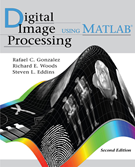# Digital Image Processing Using MATLAB: Data Types

Today's post is part of an ongoing tutorial series on digital image processing using MATLAB. I'm covering topics in roughly the order used in the book Digital Image Processing Using MATLAB.

When working with images in MATLAB, it is important to understand how different numeric data types can come into play.

The most common numeric data type in MATLAB is double, which stands for double-precision floating point. It's the representation of numbers that you get by default when you type numbers into MATLAB.

a = [0.1 0.125 1.3]

a =

0.1000    0.1250    1.3000


class(a)

ans =

double



Double-precision floating-point numbers are intended to approximate the set of real numbers. To a reasonable degree, one can do arithmetic computations on these numbers using MATLAB (and the computational hardware on your CPU or GPU) and get the same results as "true arithmetic" (or "God's math," as I've heard Cleve say) on the real numbers.

Working with floating-point numbers is very useful for mathematical image processing algorithms (such as filtering, Fourier transforms, deblurring, color computations, and many others).

Prior to 1997, the double was the only kind of data type in MATLAB. Image processing customers complained about this because of the memory required for these kinds of numbers. A double-precision floating-point number requires 64 bits, whereas many people working with image data were used to using only 8 bits (or even just 1 bit in the case of binary images) to store each pixel value.

So with MATLAB 5 and Image Processing Toolbox 2 in 1997, we introduced support for a new data type, uint8, which is an abbreviation for unsigned 8-bit integer. This data requires just 8 bits to represent a number, but the representable set of numbers is limited to the integers from 0 to 255.

You can make one in MATLAB by calling the uint8 function.

b = uint8(5)

b =

5


class(b)

ans =

uint8



Also, you often see uint8 numbers when you call the imread to read an image from a file. That's because image file formats often use 8 bits (prior to compression) to store each pixel value.

rgb = imread('peppers.png');
rgb(1:3,1:4,1)

ans =

62   63   63   65
63   61   59   64
65   63   63   66


class(rgb)

ans =

uint8



Almost immediately after MATLAB 5 and Image Processing Toolbox 2, we started hearing from customers who had scientific data stored using 16 bits for value, so 8 bits wasn't enough and 64 bits (for double) still seemed wasteful. So Image Processing Toolbox 2.2 in 1999 added support for uint16 numbers (unsigned 16-bit integers).

But still that wasn't enough. The medical imaging community, it seemed, needed signed 16-bit numbers. And, said many, what about single-precision floating-point?

For Image Processing Toolbox 3 in 2001, we stopped adding data type support piecemeal and instead added support for all the data types in MATLAB at the time. Here is a summary of the entire set:

• double - double-precision, floating-point numbers in the approximate range $\pm 10^{308}$ (8 bytes per number)
• single - single-precision, floating-point numbers with values in the approximate range $\pm 10^{38}$ (4 bytes per number)
• uint8 - unsigned 8-bit integers in the range [0,255] (1 byte per number)
• uint16 - unsigned 16-bit integers in the range [0,65535] (2 bytes per number)
• uint32 - unsigned 32-bit integers in the range [0,4294967295] (4 bytes per number)
• int8 - signed 8-bit integer in the range [-128,127] (1 byte per number)
• int16 - signed 16-bit integer in the range [-32768,32767] (2 bytes per number)
• int32 - signed 32-bit integer in the range [-2147483648,2147483647] (4 bytes per number)

Support for the logical data type (the only values are 0 and 1, 1 byte per number) was added a few years later.

Two other data types have appeared since then, uint64 and int64. Relatively little effort has been made to support these data types for image processing, for two reasons:

• We don't get any customer requests for it
• There are hard-to-answer behavior questions caused by the fact that there are uint64 and int64 numbers that can't be exactly represented as double, and some Image Processing Toolbox functions have an implicit assumption that one can convert an integer number to a double-precision floating-point number and back again without losing information in the process. But, as it turns out, there are plenty of large unsigned 64-bit numbers that can't be represented exactly in double-precision floating-point:
c = uint64(184467440737095516)

c =

184467440737095516


d = double(c)

d =

1.8447e+17


e = uint64(d)

e =

184467440737095520


e - c

ans =

4



When I pursue this topic further next time, I'll talk more about data type conversions: the basic ones in MATLAB, plus the Image Processing Toolbox ones that handle additional details of data scaling.

For more information, see Section 2.5 of Digital Image Processing Using MATLAB.Published with MATLAB® R2012b

|